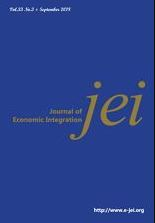상세검색
최근 검색어 전체 삭제
다국어입력
즐겨찾기0KCI등재 학술저널

# Measuring Risk of PortfolioGARCH-Copula Model

• 등재여부 : KCI등재
• 2015.03
• 172 - 205 (34 pages)
• 3

In this paper, we use the copulas functions in financial application, namely to examine the assumption of asymmetric dependence and to calculate some measures of risk. The first step consists of deducing filtered residuals for each return series by an asymmetric Glosten-Jagannathan-Runkle Generalized Autoregressive conditional Hetero skedasticity (GJR-GARCH) model. For the second step, we use an estimation of a Generalized Pareto Distribution for the upper and lower tails to determine the empirical semiparametric marginal Cumulative Distribution Function. In our approach, we propose to use a portfolio consisting of increments from five countries. The GJR-GARCH copula is then applied to the data and used to reduce correlation between the simulated residuals of each series. The marginal distributions of filtered residuals are fitted with a semi-parametric Cumulative Distribution Function using the copulas’ functions and Generalized Pareto Distribution for tails. For each series, we compute Value-at-Risk and Conditional Value-at-Risk.

Ⅰ. Introduction

Ⅱ. Review for Copula

Ⅲ. Estimation Models

Ⅳ. Empirical Analysis

Ⅴ. Conclusion